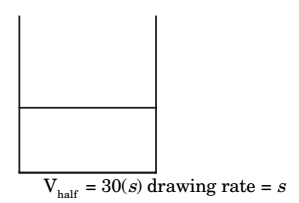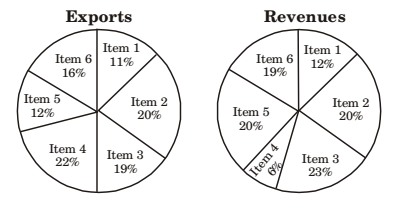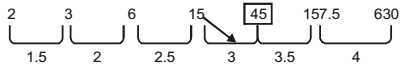## Numerical Ability

#### Numerical Ability

1. The next term in the series 81, 54, 36, 24, ...is

1.  81 – 54 = 27; 27 × 2 = 18 3

 54 – 36 = 18; 18 × 2 = 12 3

 36 – 24 = 12; 12 × 2 = 8 3

∴ 24 – 8 = 16

##### Correct Option: C

 81 – 54 = 27; 27 × 2 = 18 3

 54 – 36 = 18; 18 × 2 = 12 3

 36 – 24 = 12; 12 × 2 = 8 3

∴ 24 – 8 = 16

1. It takes 30 minutes to empty a half-full tank by draining it at a constant rate, It is decided to simultaneously pump water into the half-full tank while draining it. What is the rate at which water has to be pumped in so that it gets fully filled in 10 minutes?

1.Total volume = 60 S tank
(s1 )(10) – (s)10 = 30s
s1 (s) – s = 3s
s1 = 4s
s1 = 4 drawing rate

##### Correct Option: ATotal volume = 60 S tank
(s1 )(10) – (s)10 = 30s
s1 (s) – s = 3s
s1 = 4s
s1 = 4 drawing rate

1. The total exports and revenues from the exports of a country are given in the two charts shown below. The pie chart for exports shows the quantity of each item exported as a percentage of the total quantity of exports. The pie chart for the revenues shows the percentage of the total revenue generated through export of each item. The total quantity of exports of all the items is 500 thousand tonnes and the total revenues are 250 crore rupees. Which item among the following has generated the maximum revenue per kg?1. Item: 2

 20 × 250 × 107 100 20 × 500 × 103 100

0.5 × 104 = 5 × 103 = Item 2
Item : 3
 23 × 250 × 107 19 × 500 × 103

1.2 = Item 3
Item : 6
 19 = 1.18 = Item 6 16

Item : 5
 20 = 5 = 1.6 ⇒ 1.6 = Item 5 12 3

##### Correct Option: D

Item: 2

 20 × 250 × 107 100 20 × 500 × 103 100

0.5 × 104 = 5 × 103 = Item 2
Item : 3
 23 × 250 × 107 19 × 500 × 103

1.2 = Item 3
Item : 6
 19 = 1.18 = Item 6 16

Item : 5
 20 = 5 = 1.6 ⇒ 1.6 = Item 5 12 3

1. The sum of eight consecutive odd numbers is 656. The average of four consecutive even numbers is 87. What is the sum of the smallest odd number and second largest even number?

1. Eight consecutive odd number = 656
a – 6, a – 1, a – 2, a, a + 2, a + 4, a + 6 a + 8 = 656
a = 81 ................(1)
Smallest m = 75
Average consecutive even numbers

 ⇒ a - 2 + a + a + 2 + a + 4 = 87 4

⇒ a = 86
Second largest number = 88
1 + 2 = 163

##### Correct Option: C

Eight consecutive odd number = 656
a – 6, a – 1, a – 2, a, a + 2, a + 4, a + 6 a + 8 = 656
a = 81 ................(1)
Smallest m = 75
Average consecutive even numbers

 ⇒ a - 2 + a + a + 2 + a + 4 = 87 4

⇒ a = 86
Second largest number = 88
1 + 2 = 163

1. Fill in the missing number in the series.
2    3    6    15 _?_ 157.5    630

1.2nd number is in increasing order as shown above 1st number

##### Correct Option: A2nd number is in increasing order as shown above 1st number